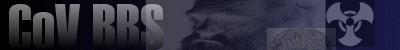Welcome, Guest. Please Login or Register. 2023-02-05 06:51:37 CoV Wiki Learn more about the Church of Virus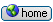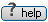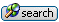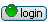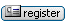News: Check out the IRC chat feature.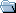Church of Virus BBS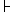General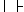Philosophy & Religion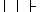Classic Texts: Lej Brouwer - Intuitionist Mathematics (1951) « previous next »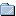Author Topic: Classic Texts: Lej Brouwer - Intuitionist Mathematics (1951)  (Read 684 times)
rhinoceros
Archon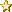Gender: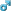Posts: 1318
Reputation: 8.42
Rate rhinocerosMy point is ...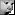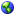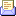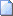Classic Texts: Lej Brouwer - Intuitionist Mathematics (1951) « on: 2003-06-18 15:56:39 »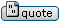Lej Brouwer

Lectures on Intuitionism (1951)
Historical introduction and Fundamental Notions

Source: Brouwer's Cambridge Lectures on Intuitionism (1951) publ. Cambridge University Press, 1981. Most of first lecture plus the appendix of fragments reproduced here.The gradual transformation of the mechanism of mathematical thought is a consequence of the modifications which, in the course of history, have come about in the prevailing philosophical ideas, firstly concerning the origin of mathematical certainty, secondly concerning the delimitation of the object of mathematical science. In this respect we can remark that in spite of the continual trend from object to subject of the place ascribed by philosophers to time and space in the subject-object medium, the belief in the existence of immutable properties of time and space, properties independent of experience and of language, remained well-nigh intact far into the nineteenth century. To obtain exact knowledge of these properties, called mathematics, the following means were usually tried: some very familiar regularities of outer or inner experience of time and space were postulated to be invariable, either exactly, or at any rate with any attainable degree of approximation. They were called axioms and put into language. Thereupon systems of more complicated properties were developed from the linguistic substratum of the axioms by means of reasoning guided by experience, but linguistically following and using the principles of classical logic. We will call the standpoint governing this mode of thinking and working the observational standpoint, and the long period characterised by this standpoint the observational period. It considered logic as autonomous, and mathematics as (if not existentially, yet functionally) dependent on logic.

For space the observational standpoint became untenable when, in the course of the nineteenth and the beginning of the twentieth centuries, at the hand of a series of discoveries with which the names of Lobatchefsky, Bolyai, Riemann, Cayley, Klein, Hilbert, Einstein, Levi-Civit&agrave; and Hahn are associated, mathematics was gradually transformed into a mere science of numbers; and when besides observational space a great number of other spaces, sometimes exclusively originating from logical speculations, with properties distinct from the traditional, but no less beautiful, had found their arithmetical realisation. Consequently the science of classical (Euclidean, three-dimensional) space had to continue its existence as a chapter without priority, on the one hand of the aforesaid (exact) science of numbers, on the other hand (as applied mathematics) of (naturally approximative) descriptive natural science.

In this process of extending the domain of geometry, an important part had been played by the logico-linguistic method, which operated on words by means of logical rules, sometimes without any guidance from experience and sometimes even starting from axioms framed independently of experience. Encouraged by this the Old Formalist School (Dedekind, Cantor, Peano, Hilbert, Russell, Zermelo, Couturat), for the purpose of a rigorous treatment of mathematics and logic (though not for the purpose of furnishing objects of investigation to these sciences), finally rejected any elements extraneous to language, thus divesting logic and mathematics of their essential difference in character, as well as of their autonomy. However, the hope originally fostered by this school that mathematical science erected according to these principles would be crowned one day with a proof of its non-contradictority was never fulfilled, and nowadays, after the logical investigations performed in the last few decades, we may assume that this hope has been relinquished universally.

Of a totally different orientation was the Pre-intuitionist School, mainly led by Poincar&eacute;, Borel and Lebesgue. These thinkers seem to have maintained a modified observational standpoint for the introduction of natural numbers, for the principle of complete induction, and for all mathematical entities springing from this source without the intervention of axioms of existence, hence for what might be called the 'separable' parts of arithmetic and of algebra. For these, even for such theorems as were deduced by means of classical logic, they postulated an existence and exactness independent of language and logic and regarded its non-contradictority as certain, even without logical proof. For the continuum, however, they seem not to have sought an origin strictly extraneous to language and logic. On some occasions they seem to have contented themselves with an ever-unfinished and ever-denumerable species of 'real numbers' generated by an ever-unfinished and ever-denumerable species of laws defining convergent infinite sequences of rational numbers. However, such an ever-unfinished and ever-denumerable species of 'real numbers' is incapable of fulfilling the mathematical function of the continuum for the simple reason that it cannot have a positive measure. On other occasions they seem to have introduced the continuum by having recourse to some logical axiom of existence, such as the 'axiom of ordinal connectedness', or the 'axiom of completeness', without either sensory or epistemological evidence. In both cases in their further development of mathematics they continued to apply classical logic, including the principium tertii exclusi, without reserve and independently of experience. This was done regardless of the fact that the noncontradictority of systems thus constructed had become doubtful by the discovery of the well-known logico-mathematical antonomies.

In point of fact, pre-intuitionism seems to have maintained on the one hand the essential difference in character between logic and mathematics, and on the other hand the autonomy of logic, and of a part of mathematics. The rest of mathematics became dependent on these two.

Meanwhile, under the pressure of well-founded criticism exerted upon old formalism, Hilbert founded the New Formalist School, which postulated existence and exactness independent of language not for proper mathematics but for meta-mathematics, which is the scientific consideration of the symbols occurring in perfected mathematical language, and of the rules of manipulation of these symbols. On this basis new formalism, in contrast to old formalism, in confesso made primordial practical use of the intuition of natural numbers and of complete induction. It is true that only for a small part of mathematics (much smaller than in pre-intuitionism) was autonomy postulated in this way. New formalism was not deterred from its procedure by the objection that between the perfection of mathematical language and the perfection of mathematics itself no clear connection could be seen.

So the situation left by formalism and pre-intuitionism can be summarised as follows: for the elementary theory of natural numbers, the principle of complete induction and more or less considerable parts of arithmetic and of algebra, exact existence, absolute reliability and non-contradictority were universally acknowledged, independently of language and without proof. As for the continuum, the question of its languageless existence was neglected, its establishment as a set of real numbers with positive measure was attempted by logical means and no proof of its non-contradictory existence appeared. For the whole of mathematics the four principles of classical logic were accepted as means of deducing exact truths.

In this situation intuitionism intervened with two acts, of which the first seems to lead to destructive and sterilising consequences, but then the second yields ample possibilities for new developments.

FIRST ACT OF INTUITIONISM

Completely separating mathematics from mathematical language and hence from the phenomena of language described by theoretical logic, recognising that intuitionistic mathematics is an essentially languageless activity of the mind having its origin in the perception of a move of time. This perception of a move of time may be described as the falling apart of a life moment into two distinct things, one of which gives way to the other, but is retained by memory. If the twoity thus born is divested of all quality, it passes into the empty form of the common substratum of all twoities. And it is this common substratum, this empty form, which is the basic intuition of mathematics.

Inner experience reveals how, by unlimited unfolding of the basic intuition, much of 'separable' mathematics can be rebuilt in a suitably modified form. In the edifice of mathematical thought thus erected, language plays no part other than that of an efficient, but never infallible or exact, technique for memorising mathematical constructions, and for communicating them to others, so that mathematical language by itself can never create new mathematical systems. But because of the highly logical character of this mathematical language the following question naturally presents itself. Suppose that, in mathematical language, trying to deal with an intuitionist mathematical operation, the figure of an application of one of the principles of classical logic is, for once, blindly formulated. Does this figure of language then accompany an actual languageless mathematical procedure in the actual mathematical system concerned?

A careful examination reveals that, briefly expressed, the answer is in the affirmative, as far as the principles of contradiction and syllogism are concerned,' if one allows for the inevitable inadequacy of language as a mode of description and communication. But with regard to the principle of the excluded third, except in special cases, the answer is in the negative, so that this principle cannot in general serve as an instrument for discovering new mathematical truths.

Indeed, if each application of the principium tertii exclusi in mathematics accompanied some actual mathematical procedure, this would mean that each mathematical assertion (i.e. an assignment of a property to a mathematical entity) could be judged, that is to say could either be proved or be reduced to absurdity.

Now every construction of a bounded finite nature in a finite mathematical system can only be attempted in a finite number of ways, and each attempt proves to be successful or abortive in a finite number of steps. We conclude that every assertion of possibility of a construction of a bounded finite nature in a finite mathematical system can be judged, so that in these circumstances applications of the Principle of the Excluded Third are legitimate.

But now let us pass to infinite systems and ask for instance if there exists a natural number n such that in the decimal expansion of pi the nth, (n+1)th, ..., (n+8)th and (n+9)th digits form a sequence 0123456789. This question, relating as it does to a so far not judgeable assertion, can be answered neither affirmatively nor negatively. But then, from the intuitionist point of view, because outside human thought there are no mathematical truths, the assertion that in the decimal expansion of pi a sequence 0123456789 either does or does not occur is devoid of sense.

The aforesaid property, suppositionally assigned to the number n, is an example of a fleeing property, by which we understand a property f, which satisfies the following three requirements:

(i) for each natural number n it can be decided whether or not n possesses the property f,

(ii) no way of calculating a natural number n possessing f is known;

(iii) the assumption that at least one natural number possesses f is not known to be an absurdity.

Obviously the fleeing nature of a property is not necessarily permanent, for a natural number possessing f might at some time be found, or the absurdity of the existence of such a natural number might at some time be proved.

...

The belief in the universal validity of the principle of the excluded third in mathematics is considered by the intuitionists as a phenomenon of the history of civilization of the same kind as the former belief in the rationality of pi, or in the rotation of the firmament about the earth. The intuitionist tries to explain the long duration of the reign of this dogma by two facts: firstly that within an arbitrarily given domain of mathematical entities the non-contradictority of the principle for a single assertion is easily recognized; secondly that in studying an extensive group of simple every-day phenomena of the exterior world, careful application of the whole of classical logic was never found to lead to error. [This means de facto that common objects and mechanisms subjected to familiar manipulations behave as if the system of states they can assume formed part of a finite discrete set, whose elements are connected by a finite number of relations.]

The mathematical activity made possible by the first act of intuitionism seems at first sight, because mathematical creation by means of logical axioms is rejected, to be confined to 'separable' mathematics, mentioned above; while, because also the principle of the excluded third is rejected, it would seem that even within 'separable' mathematics the field of activity would have to be considerably curtailed. In particular, since the continuum appears to remain outside its scope, one might fear at this stage that in intuitionism there would be no place for analysis. But this fear would have assumed that infinite sequences generated by the intuitionistic unfolding of the basic intuition would have to be fundamental sequences, i.e. predeterminate infinite sequences proceeding, like classical ones, in such a way that from the beginning the nth term is fixed for each n. Such however is not the case; on the contrary, a much woder field of development, including analysis and often exceeding the frontiers of classical mathematics, is opened by the second act of intuitionism.

SECOND ACT OF INTUITIONISM

Admitting two ways of creating new mathematical entities: firstly in the shape of more or less freely proceeding infinite sequences of mathematical entities previously acquired (so that, for decimal fractions having neither exact values, not any guarantee of ever getting exact values admitted); secondly in the shape of mathematical species, i.e. properties supposable for mathematical entities previously acquired, satisfying the condition that if they hold for a certain mathematical entity, they also hold for all mathematical entities which have been defined to be 'equal' to it, definitions of equality having to satisfy the conditions of symmetry, relfexivity and transitivity. ...

Theorems holding in intuitionistic, but not in classical, mathematics often originate from the circumstance that for mathematical entities belonging to a certain species the inculcation of a certain property imposes a special character on their way of development from the basic intuition; and that from this compulsory special character properties ensue which for classical mathematics are false. Striking examples are the modern theorems that the continuum does not split, and that a full function of the unit continuum is necessarily uniformly continuous.

Notes

Introvert science, directed at beauty, does not carry risks for consequences.

The stock of mathematical entities is a real thing, for each person, and for humanity.

The inner experience (roughly sketched):

• twoity;
• twoity stored and preserved aseptically by memory;
• twoity giving rise to the conception of invariable unity;
• twoity and unity giving rise to the conception of unity plus unity;
• threeity as twoity plus unity, and the sequence of natural numbers;
• mathematical systems conceived in such a way that a unity is a mathematical system and that two mathematical systems, stored and aseptically preserved by memory, apart from each other, can be added;

etc.

Fragments from a lecture 'Changes in the relation between classical logic and mathematics. (The influence of intuitionistic mathematics on logic)', given in November 1951

Classical logic presupposed that independently of human thought there is a truth, part of which is expressible by means of sentences called 'true assertions', mainly assigning certain properties to certain objects or stating that objects possessing certain properties exist or that certain phenomena behave according to certain laws. Furthermore classical logic assumed the existence of general linguistic rules allowing an automatic deduction of new true assertions from old ones, so that starting from a limited stock of 'evidently' true assertions, mainly founded on experience and called axioms, an extensive supplement to existing human knowledge would theoretically be accessible by means of linguistic operations independently of experience. Finally, using the term 'false' for the 'converse of true', classical logic assumed that in virtue of the so-called 'principle of the excluded third' each assertion, in particular each existence assertion and each assignment of a property to an object or of a behaviour to a phenomenon, is either true or false independently of human beings knowing about this falsehood or truth, so that, for example, contradictorily of falsehood would imply truth whilst an assertion a which is true if the assertion b is either true or false would be universally true. The principle holds if 'true' is replaced by 'known and registered to be true', but then this classification is variable, so that to the wording of the principle we should add 'at a certain moment'.

As long as mathematics was considered as the science of space and time, it was a beloved field of activity of this classical logic, not only in the days when space and time were believed to exist independently of human experience, but still after they had been taken for innate forms of conscious exterior human experience. There continued to reign some conviction that a mathematical assertion is either false or true, whether we know it or not, and that after the extinction of humanity mathematical truths, just as laws of nature, will survive. About half a century ago this was expressed by the great French mathematician Charles Hermite in the following words: 'Il existe, si je ne me trompe, tout un monde qui est l'ensemble des v&eacute;rit&eacute;s math&eacute;matiques, dans lequel nous n'avons d'acc&eacute;s que par l'intelligence, comme existe le monde des r&eacute;alit&eacute;s physiques; l'un et l'autre ind&eacute;pendant de nous, tous deux de cr&eacute;ation divine et qui ne semblent distincts qu&agrave; cause de la faiblesse de notre esprit, par contre ne sont pour une pens&eacute;e puissante qu'une seule et m&ecirc;me chose, et dont la synth&egrave;se se r&eacute;l&egrave;ve partiellement dans cette merveilleuse correspondence entre les Math&eacute;matiques abstraites d'une part, I'Astronomie, et toutes les branches de la Physique de I'autre'.

Only after mathematics had been recognized as an autonomous interior constructional activity which, although it can be applied to an exterior world, neither in its origin nor in its methods depends on an exterior world, firstly all axioms became illusory, and secondly the criterion of truth or falsehood of a mathematical assertion was confined to mathematical activity itself, without appeal to logic or to hypothetical omniscient beings. An immediate consequence was that for a mathematical assertion a the two cases of truth and falsehood, formerly exclusively admitted, were replaced by the following three:

(1) a has been proved to be true;

(2) a has been proved to be absurd;

(3) a has neither been proved to be true nor to be absurd, nor do we know a finite algorithm leading to the statement either that a is true or that a is absurd. [The case that a has neither been proved to be true nor to be absurd, but that we know a finite algorithm leading to the statement either that a is true, or that a is absurd, obviously is reducible to the first and second cases. This applies in particular to assertions of possibility of a construction of bounded finite character in a finite mathematical system, because such a construction can be attempted only in a finite number of particular ways, and each attempt proves successful or abortive in a finite number of steps.]

In contrast to the perpetual character of cases (1) and (2), an assertion of type (3) may at some time pass into another case, not only because further thinking may generate an algorithm accomplishing this passage, but also because in modern or intuitionistic mathematics, as we shall see presently, a mathematical entity is not necessarily predeterminate, and may, in its state of free growth, at some time acquire a property which it did not possess before.

See lecture above on fleeing property

One of the reasons [incorrect, the extension is an immediate consequence of the self-unfolding; so here only the utility of the extension is explained.] that led intuitionistic mathematics to this extension was the failure of classical mathematics to compose the continuum out of points without the help of logic. For, of real numbers determined by predeterminate convergent infinite sequences of rational numbers, only an ever-unfinished denumerable species can actually be generated. This ever-unfinished denumerable species being condemned never to exceed the measure zero, classical mathematics, in order to compose a continuum of positive measure out of points, has recourse to some logical process starting from at least an axiom. A rather common method of this kind is due to Hilbert who, starting from a set of properties of order and calculation, including the Archimedean property, holding for the arithmetic of the field of rational numbers, and considering successive extensions of this field and arithmetic to the extended fields and arithmetics conserving the foresaid properties, including the preceding fields and arithmetics, postulates the existence of an ultimate such extended field and arithmetic incapable of further extension, i.e. he applies the so-called axiom of completeness. From the intuitionistic point of view the continuum created in this way has a merely linguistic, and no mathematical, existence. It is only by means of the admission of freely proceeding infinite sequences that intuitionistic mathematics has succeeded to replace this linguistic continuum by a genuine mathematical continuum of positive measure, and the linguistic truths of classical analysis by genuine mathematical truths.

However, notwithstanding its rejection of classical logic as an instrument to discover mathematical truths, intuitionistic mathematics has its general introspective theory of mathematical assertions. This theory, which with some right may be called intuitionistic mathematical logic, we shall illustrate by the following remarks.

 « Last Edit: 2003-06-18 16:56:27 by rhinoceros » Report to moderator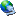Logged
 Jump to:Please select a destination: ----------------------------- General ----------------------------- =>Announcements =>Evolution and Memetics =>FAQ =>Church Doctrine =>Science & Technology =>Society & Culture =>Arts & Entertainment =>Philosophy & Religion =>Humor & Satire =>Creative Endeavors =>Serious Business =>Virian Book Club =>Second Life =>Free For All =>Infections =>Suggestion Box =>Test Area ----------------------------- Mailing List ----------------------------- =>Virus 2006 =>Virus 2005 =>Virus 2004 =>Virus 2003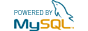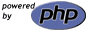Church of Virus BBS | Powered by YaBB SE © 2001-2002, YaBB SE Dev Team. All Rights Reserved. Please support the CoV.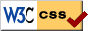RSS feed